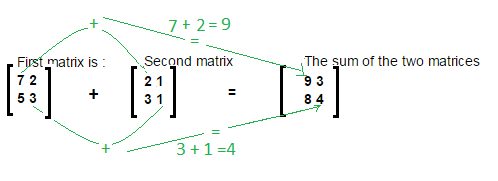### Matrix Addition program in java

Hi! we will learn how to add two matrices in java.
Both matrices must have same number of rows and columns.

Let’s understand addition of matrices by diagram.Main logic behind Matrix Addition is:
//Subtraction of matrices.
int[][] resultMatix = new int[rows][columns];
for (int i = 0; i < rows; i++) {
for (int j = 0; j < columns; j++) {
resultMatix[i][j] = matrix1[i][j] + matrix2[i][j];
}
}

Example/ Full Program/SourceCode for Matrix Addition in java >
 package matrix; import java.util.Scanner; /** * @author AnkitMittal */ /** Copyright (c), AnkitMittal  JavaMadeSoEasy.com - Matrix Addition in java*/ public class MatrixAddition {    public static void main(String...args) {           Scanner scanner = new Scanner(System.in);           System.out.print("Enter number of rows in matrix : "); //rows and columns in matrix1 and matrix2 must be same for addition.           int rows = scanner.nextInt();           System.out.print("Enter number of columns in matrix : ");           int columns = scanner.nextInt();           int[][] matrix1 = new int[rows][columns];           int[][] matrix2 = new int[rows][columns];                     System.out.println("Enter the elements in first matrix :");           for (int i = 0; i < rows; i++) {                  for (int j = 0; j < columns; j++) {                        matrix1[i][j] = scanner.nextInt();                  }           }           System.out.println("Enter the elements in second matrix :");           for (int i = 0; i < rows; i++) {                  for (int j = 0; j < columns; j++) {                        matrix2[i][j] = scanner.nextInt();                  }           }                     //addition of matrices.           int[][] resultMatix = new int[rows][columns];           for (int i = 0; i < rows; i++) {                  for (int j = 0; j < columns; j++) {                        resultMatix[i][j] = matrix1[i][j] + matrix2[i][j];                  }           }           System.out.println("\nFirst matrix is : ");           for (int i = 0; i < rows; i++) {                  for (int j = 0; j < columns; j++) {                        System.out.print(matrix1[i][j] + " ");                  }                  System.out.println();           }           System.out.println("\nSecond matrix is : ");           for (int i = 0; i < rows; i++) {                  for (int j = 0; j < columns; j++) {                        System.out.print(matrix2[i][j] + " ");                  }                  System.out.println();           }           System.out.println("\nThe sum of the two matrices is : ");           for (int i = 0; i < rows; i++) {                  for (int j = 0; j < columns; j++) {                        System.out.print(resultMatix[i][j] + " ");                  }                  System.out.println();           }    } } /*OUTPUT Enter number of rows in matrix : 2 Enter number of columns in matrix : 2 Enter the elements in first matrix : 7 2 5 3 Enter the elements in second matrix : 2 1 3 1 First matrix is : 7 2 5 3 Second matrix is : 2 1 3 1 The sum of the two matrices is : 9 3 8 4 */

So we learned program to do Matrix Addition in java.

Having any doubt? or you you liked the tutorial! Please comment in below section.

>Matrix Addition in java